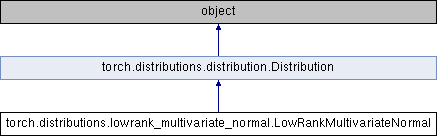Caffe2 - Python API A deep learning, cross platform ML framework
torch.distributions.lowrank_multivariate_normal.LowRankMultivariateNormal Class Reference
Inheritance diagram for torch.distributions.lowrank_multivariate_normal.LowRankMultivariateNormal:## Public Member Functions

def __init__ (self, loc, cov_factor, cov_diag, validate_args=None)

def expand (self, batch_shape, _instance=None)

def mean (self)

def variance (self)

def scale_tril (self)

def covariance_matrix (self)

def precision_matrix (self)

def rsample (self, sample_shape=torch.Size())

def log_prob (self, value)

def entropy (self)Public Member Functions inherited from torch.distributions.distribution.Distribution
def __init__ (self, batch_shape=torch.Size(), event_shape=torch.Size(), validate_args=None)

def expand (self, batch_shape, _instance=None)

def batch_shape (self)

def event_shape (self)

def arg_constraints (self)

def support (self)

def mean (self)

def variance (self)

def stddev (self)

def sample (self, sample_shape=torch.Size())

def rsample (self, sample_shape=torch.Size())

def sample_n (self, n)

def log_prob (self, value)

def cdf (self, value)

def icdf (self, value)

def enumerate_support (self, expand=True)

def entropy (self)

def perplexity (self)

def __repr__ (self)

loc

cov_diag

## Static Public Attributes

arg_constraints

support

has_rsampleStatic Public Attributes inherited from torch.distributions.distribution.Distribution
has_rsample

has_enumerate_support

support

arg_constraintsStatic Public Member Functions inherited from torch.distributions.distribution.Distribution